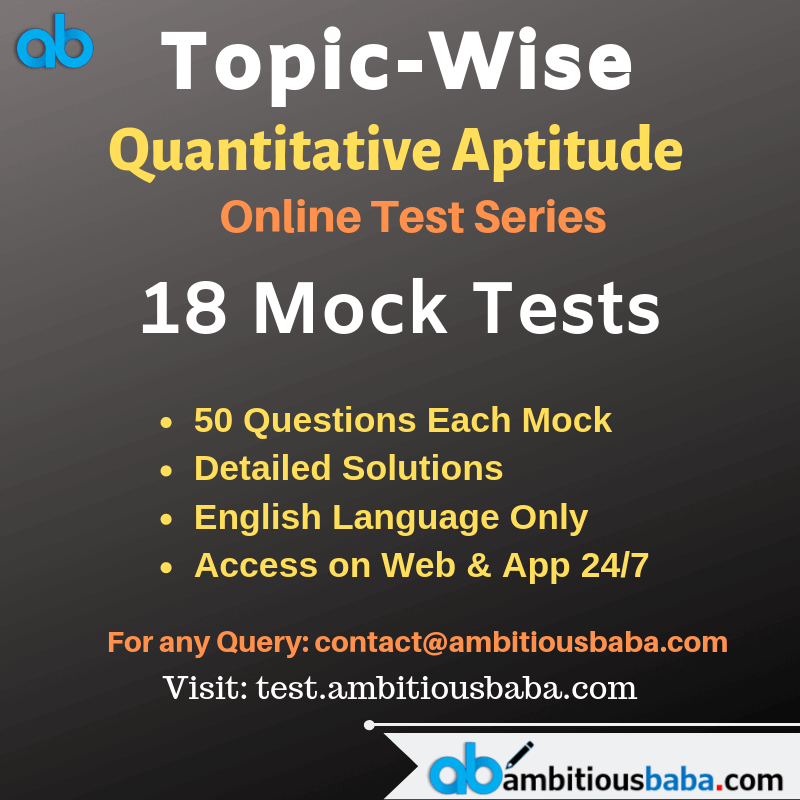# Quant Quiz for IBPS CLERK MAINS 2020 |4 January 2020

## Quant Quiz for IBPS CLERK MAINS:

Quant Quiz to improve your Quantitative Aptitude for SBI Po & SBI clerk exam IBPS PO Reasoning , IBPS Clerk Reasoning , IBPS RRB Reasoning, LIC AAO ,LIC Assistant  and other competitive exams

Q1. Given that D is the midpoint of AC and BC is diameter of circle, and circumference of circle is 44 cm.
Quantity I- area of shaded region
Quantity II-(A) Quantity I > Quantity II

(B) Quantity I < Quantity II

(c) Quantity I ≥ Quantity II

(D) Quantity I ≤ Quantity II

(E) Quantity I = Quantity II or no relation

Q2.(A) Quantity I > Quantity II

(B) Quantity I < Quantity II

(c) Quantity I ≥ Quantity II

(D) Quantity I ≤ Quantity II

(E) Quantity I = Quantity II or no relation

Q3.  Speed of a boat in still water and speed of current is in ratio 6 : 1. If the difference between distance covered by boat in 2 hours upstream and in 2 hours downstream is 8 km.
Quantity-1- Speed of boat in still water
Quantity-2- speed of cyclist who goes 28 km in 2 hrs.

(A) Quantity I > Quantity II

(B) Quantity I < Quantity II

(c) Quantity I ≥ Quantity II

(D) Quantity I ≤ Quantity II

(E) Quantity I = Quantity II or no relation

Q4. Quantity I — cost price of an article having marked price = Rs. 400, which when sold at 20% discount still make a gain ofQuantity II — cost price of an article which is sold at 14% profit and if cost price and selling price both are Rs. 117 less, the profit would be 9% more.

(A) Quantity I > Quantity II

(B) Quantity I < Quantity II

(c) Quantity I ≥ Quantity II

(D) Quantity I ≤ Quantity II

(E) Quantity I = Quantity II or no relation

Q5.  Quantity I — the sum of money for which the difference between SI and CI obtained on it in 2 years at 6% per annum compounded annually is Rs. 43.2.
Quantity II — Rs. 12850

(A) Quantity I > Quantity II

(B) Quantity I < Quantity II

(c) Quantity I ≥ Quantity II

(D) Quantity I ≤ Quantity II

(E) Quantity I = Quantity II or no relation

Q6.  Quantity I — average income of the whole group of 75 people, if average income of the men in the group is Rs. 4200 and that of women is Rs. 4000. (total men : total women = 8 : 7)
Quantity II — The average income of 20 people, which decreases by Rs. 150 if a person with income of Rs. 1000 joins them.

(A) Quantity I > Quantity II

(B) Quantity I < Quantity II

(c) Quantity I ≥ Quantity II

(D) Quantity I ≤ Quantity II

(E) Quantity I = Quantity II or no relation

Q7. Quantity I — The distance of school from Aman’s house if he reaches school 5 minutes late which walking at 4 km/hr but 10 minutes earlier than scheduled time walking at 5 km/hr.
Quantity II — 5 km

(A) Quantity I > Quantity II

(B) Quantity I < Quantity II

(c) Quantity I ≥ Quantity II

(D) Quantity I ≤ Quantity II

(E) Quantity I = Quantity II or no relation

Q8.  Product of 2 numbers, whose sum is 17 and sum of the squares of 2 no. is 145.
Quantity II — Sum of 2 numbers, whose product is 1400 and difference between them is 5.

(A) Quantity I > Quantity II

(B) Quantity I < Quantity II

(c) Quantity I ≥ Quantity II

(D) Quantity I ≤ Quantity II

(E) Quantity I = Quantity II or no relation

Q9.  Quantity I.The average of the five consecutive odd numbers is 23. Then, find the difference between the square of largest and smallest odd numbers.
Quantity II.
Find the value of x (Use approximation)
495.01 + 63.98% of 949.963 = 738.0 + x

(A) Quantity I > Quantity II

(B) Quantity I < Quantity II

(c) Quantity I ≥ Quantity II

(D) Quantity I ≤ Quantity II

(E) Quantity I = Quantity II or no relation

Q10.(A) Quantity I > Quantity II

(B) Quantity I < Quantity II

(c) Quantity I ≥ Quantity II

(D) Quantity I ≤ Quantity II

(E) Quantity I = Quantity II or no relation

## Solutions

Q1. Ans(A)Q2. Ans(B)Q3. Ans(B)Q4. Ans(A)Q5. Ans(B)Q6. Ans(B)Q7. Ans(E)Q8. Ans(E)Q9. Ans(A)Q10. Ans(C)### Online Mock Test Available on App as well as Web:### How to Access on App:-

1. Go to Playstore search Ambitious Baba or Click here to Install App

### You Can Read This Also:

ambitiousbaba.com need your support to Grow

I challenge you will get Best Content in Our PDFs with Detail solutions and Latest Pattern

Memory Based Puzzle E-book | 2016-19 Exams Covered

Get PDF here

Caselet Data Interpretation 200 Questions

Get PDF here
Puzzle & Seating Arrangement E-Book for BANK PO MAINS (Vol-1)

Get PDF here

### ARITHMETIC DATA INTERPRETATION 2019 E-book

Get PDF here
The Banking Awareness 500 MCQs E-book| Bilingual (Hindi + English)

Get PDF here

High Level DATA INTERPRETATION Practice E-BOOK

Get PDF her

3